@zhuanxu 2018-01-24T03:41:15.000000Z 字数 2886 阅读 8369

# 贝叶斯统计：Gibbs sampling 原理到实践

贝叶斯 lda gibbs

## Gibbs采样

Gibbs采样的算法如下：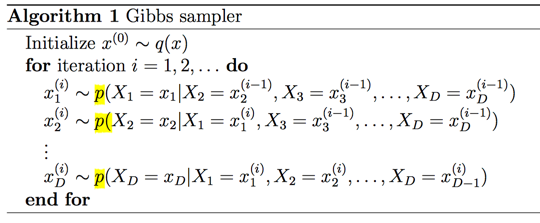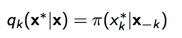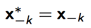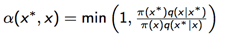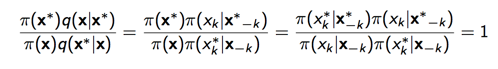$\pi(x^*)=\pi(x_{k}^{*}|x_{-k}^{*})\pi(x_{-k}^{*})$,
$\pi(x)=\pi(x_{k}|x_{-k})\pi(x_{-k})$

## 例子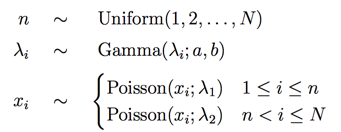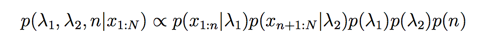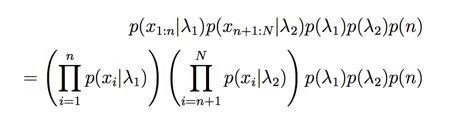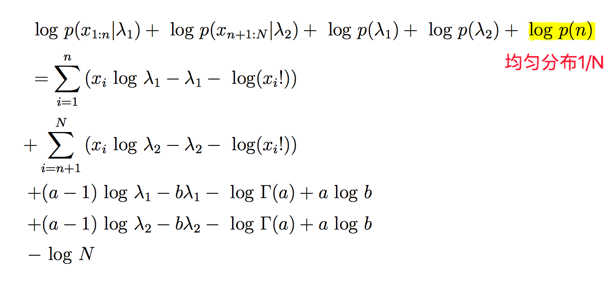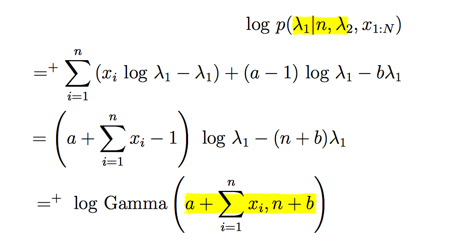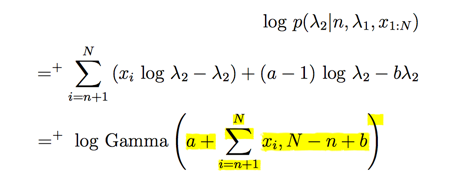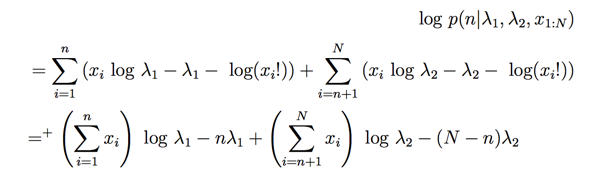n没有显示的分布信息，但是我们简单将其认为是一个多项分布。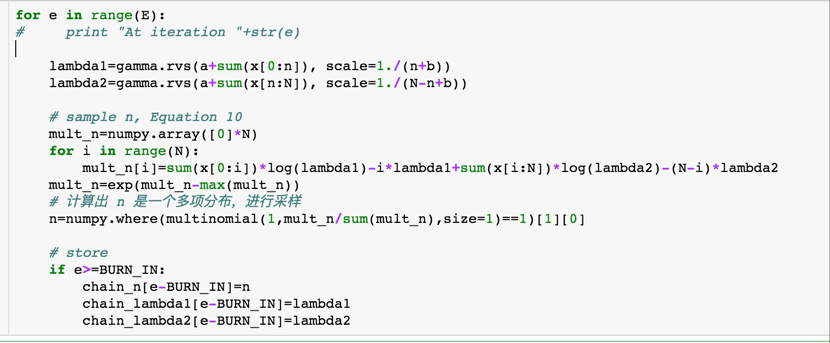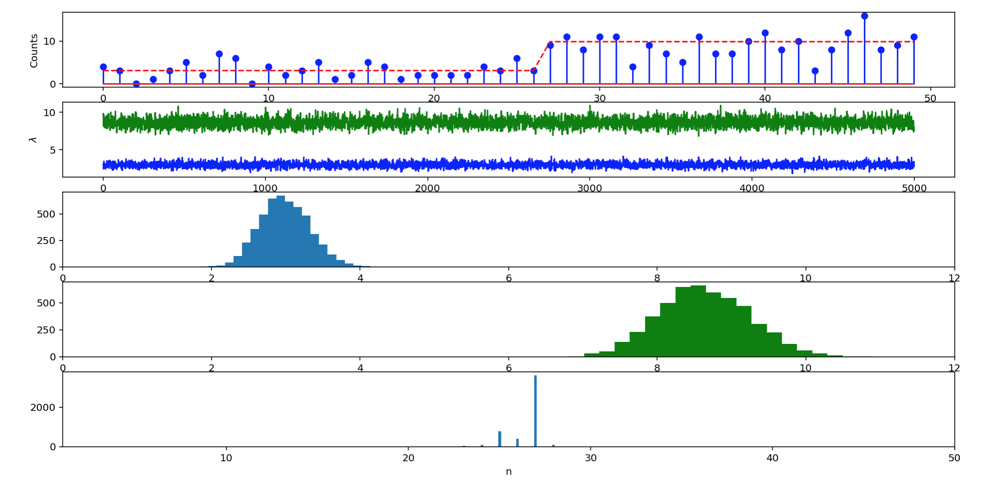## LDA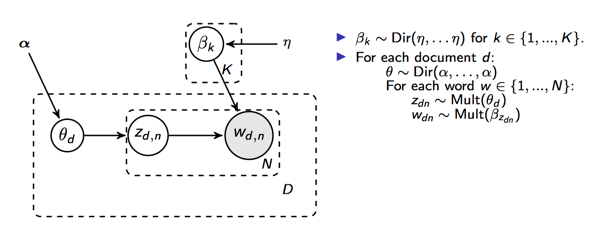1. 对于文档d，从$Dir(\alpha)$分布中采样出$\theta_d$，即文档d的主题分布
2. 对于文档的每个词$w_{d,n}$从多项分布$\theta_d$中采样出主题k，即$z_{d,n}=k$
3. 对于主题k，从$Dir(\eta)$中采样出$\beta_k$，即主题k的词分布
4. 对于每个词$w_{d,n}$，从主题分布$\beta_{z_{d,n}}$中采样出具体的单词t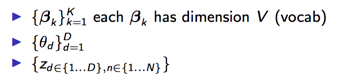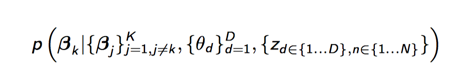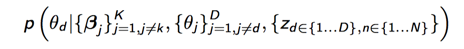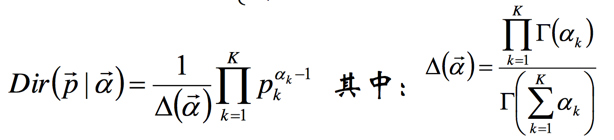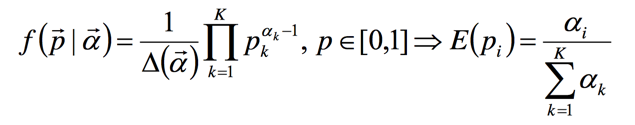## 编码

for m in range(D): // 遍历每篇文档    for n in range(W[m]): // 遍历每篇文档的单词        w = W[m][n] // 单词 w        k = z[m][n] // 主题 k        nd[m,k] -= 1 // 第m篇文档第k个主题的词数目        ndsum[m, 0] -= 1 // 第m篇文档总的主题词数目sum(nd[m])        nw[k, w] -= 1 // 第k个topic中产生w的数目        nwsum[k, 0] -= 1 // 第k个topic总共产生的w数目        # 计算theta值，Dir分布的期望        theta_p = (nd[m] + alpha) / (ndsum[m, 0] + sum_alpha)        # 计算beta的期望值        beta_p = (nw[:, w] + beta[w]) / (nwsum[:, 0] + sum_beta)        # multi_p为多项式分布的参数        multi_p = theta_p * beta_p        # 多项式采样新的k        k = mul_sample(multi_p)        nd[m,k] += 1 // 第m篇文档第k个主题的词数目        ndsum[m, 0] += 1 // 第m篇文档总的主题词数目sum(nd[m])        nw[k, w] += 1 // 第k个topic中产生w的数目        nwsum[k, 0] += 1 // 第k个topic总共产生的w数目        z[m][n] = k// 更新主题

## pymc3 实现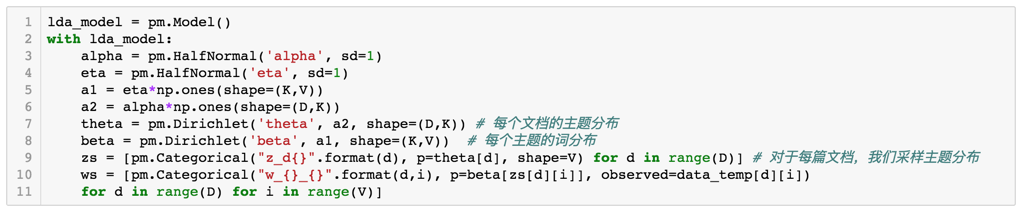## 总结• 私有
• 公开
• 删除